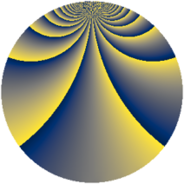# Properties

 Label 920.2.bfLevel $920$ Weight $2$ Character orbit 920.bf Rep. character $\chi_{920}(29,\cdot)$ Character field $\Q(\zeta_{22})$ Dimension $1400$ Newform subspaces $1$ Sturm bound $288$ Trace bound $0$

# Related objects

## Defining parameters

 Level: $$N$$ $$=$$ $$920 = 2^{3} \cdot 5 \cdot 23$$ Weight: $$k$$ $$=$$ $$2$$ Character orbit: $$[\chi]$$ $$=$$ 920.bf (of order $$22$$ and degree $$10$$) Character conductor: $$\operatorname{cond}(\chi)$$ $$=$$ $$920$$ Character field: $$\Q(\zeta_{22})$$ Newform subspaces: $$1$$ Sturm bound: $$288$$ Trace bound: $$0$$

## Dimensions

The following table gives the dimensions of various subspaces of $$M_{2}(920, [\chi])$$.

Total New Old
Modular forms 1480 1480 0
Cusp forms 1400 1400 0
Eisenstein series 80 80 0

## Trace form

 $$1400q - 14q^{4} - 14q^{6} - 168q^{9} + O(q^{10})$$ $$1400q - 14q^{4} - 14q^{6} - 168q^{9} - 5q^{10} - 26q^{14} - 6q^{15} - 14q^{16} - 27q^{20} - 32q^{24} - 18q^{25} - 30q^{26} - q^{30} - 52q^{31} + 40q^{34} + 26q^{36} - 12q^{39} + 15q^{40} - 36q^{41} - 142q^{44} - 10q^{46} + 80q^{49} - 47q^{50} + 46q^{54} - 38q^{55} + 14q^{56} + 21q^{60} - 26q^{64} + 2q^{65} - 104q^{66} - 26q^{70} - 36q^{71} - 18q^{74} - 96q^{76} - 12q^{79} + 82q^{80} - 136q^{81} - 82q^{84} - 198q^{86} - 52q^{89} + 164q^{90} - 38q^{94} + 2q^{95} - 432q^{96} + O(q^{100})$$

## Decomposition of $$S_{2}^{\mathrm{new}}(920, [\chi])$$ into newform subspaces

Label Dim. $$A$$ Field CM Traces $q$-expansion
$$a_2$$ $$a_3$$ $$a_5$$ $$a_7$$
920.2.bf.a $$1400$$ $$7.346$$ None $$0$$ $$0$$ $$0$$ $$0$$